# Device Electrical Characteristics

### Overview

When designing an embedded system, designers must evaluate if two components are electrically compatible with each other.  Two devices are electrically compatible with one another if both devices can provide appropriate voltage levels for logic 0 and logic 1.  We will examine how to determine if two devices are compatible and also state how tolerant a system is to transient noise on a signal.

### Logic Levels

A digital pin interprets analog voltages as either logic 0 or logic 1.   So what voltage values correspond to logic 0 and logic 1?  The answer to that depends on the manufacturing technology and the supply voltage used to power a device.  The most common manufacturing technology used in modern electronics is TTL or transistor to transistor logic.  Depending on the sub-family, TTL logic most commonly supports voltage ranges from 1.65V to 3.3V.

The microcontroller used in class uses TTL logic that supports a supply voltage from 3.15V to 3.63V.  It also states the the nominal voltage should be 3.3V.  The nominal voltage is simply the voltage that the device was designed to operate at.  In almost all circumstances we will design our embedded systems to work at the nominal voltage level.

So if a IO pin is configured as an input and a voltage level of 3.3V is placed on that pin, the IO pin will interpret that voltage as logic 1.  If 0.0V was placed on the same pin, this voltage value is interpreted as logic 0.  But what happens if a voltage of 3.1V is placed on the same pin?  Does the voltage represent a logic 0 or a logic 1?  To answer that question “Read the datasheet”.

Any modern device is going to have a chapter called Electrical Characteristics or something very similar.  This chapter describes the voltage levels and nominal operating conditions for a device.  We can examine this chapter to determine what voltage levels represent logic 0 and logic 1.

The table below was taken from the TM4C123GH6PM data sheet, page 1361.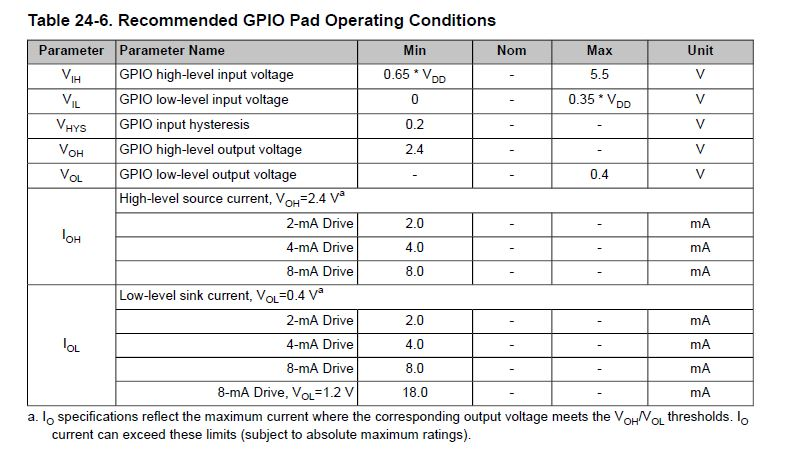#### Input Considerations

Since we are considering the situation where a IO pins is configured as an input, we are concerned with the first two rows of the table.  The first row has a named parameter VIH.  This parameter indicates the voltage levels required for a logic 1.

There are two columns that are important to VIH, the Min and Max columns.  The Min column tells us that the minimum voltage that will be interpreted as logic 1 is 0.65 of the supply voltage.  If we assume the supply voltage is 3.3V, then this number is 2.145V.  This value is referred to as VIHMIN, or the minimum value that can represent logic 1 when a pin is configured as an input.

The max column tells us what is the maximum voltage that can be supplied to the pin without damaging the part.  This value is 5.5V.  This means that the pin is considered to be 5V tolerant since a 5V input will not damage the part.

The second row in the table gives us information on logic 0 or VIL.  The minimum voltage that can be placed on a IO pin is 0.0V.  The maximum voltage that is still interpreted as logic 0 is 0.35 of the supply voltage, or 1.155V. This voltage is referred to as VILMAX or the maximum value that can represent logic 0 when a pin is configured as an input.

The diagram below visualizes the voltages that are interpreted as logic 0 and 1 when a IO pin is configured as an input.  Any voltage greater than VIHMIN will be interpreted as logic 1.  Any voltage less than VILMAX will be interpreted as logic 0.  A voltage in between these values, say 1.5V, is essentially unknown.  As a designer, we cannot predict what logic value this voltage will be interpreted as.  This is an invalid voltage input and will most likely cause a system to malfunction.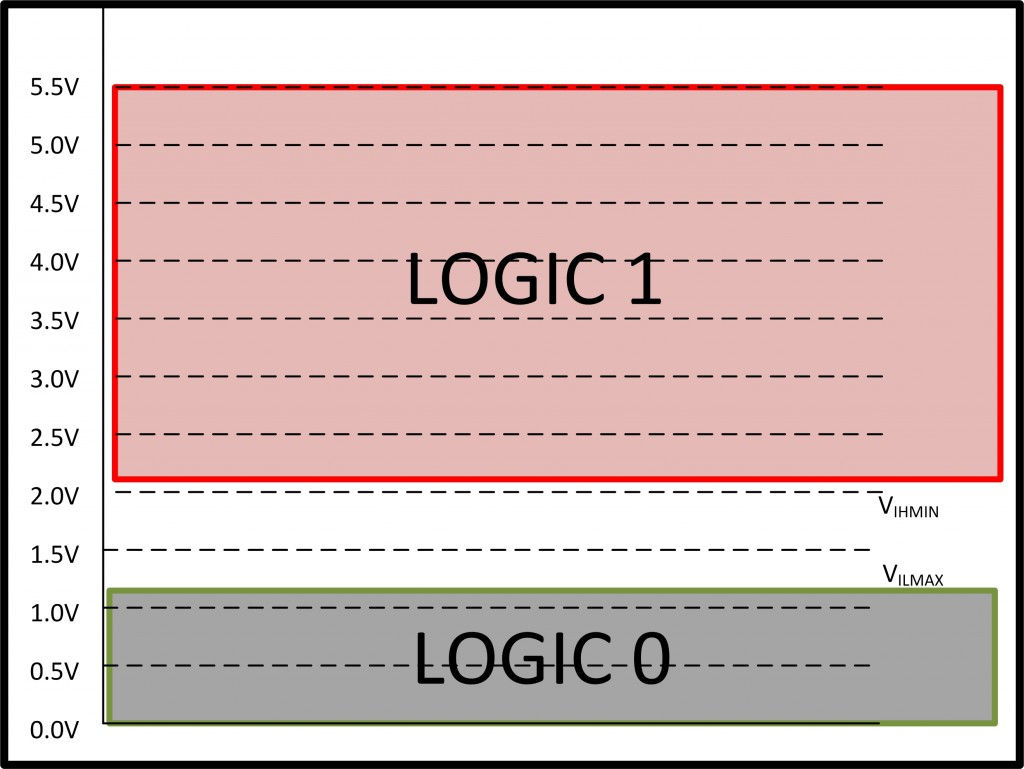#### Output Considerations

We now will examine a IO pin configured as an output.  We want to determine what voltage level the TM4C123 will place on one of its IO pins for logic 0 and logic 1.

Row 4 tells us that if the supply voltage is 3.3V, a logic value of 1 will generate a voltage level on the specified pin of 2.4V or greater.  This value is referred to as the VOHMIN.

Row 5 tells us that if the supply voltage is 3.3V, a logic value of 0 will be guaranteed to generated a voltage level on the specified pin less than 0.4V.   This value is referred to as the VOLMAX.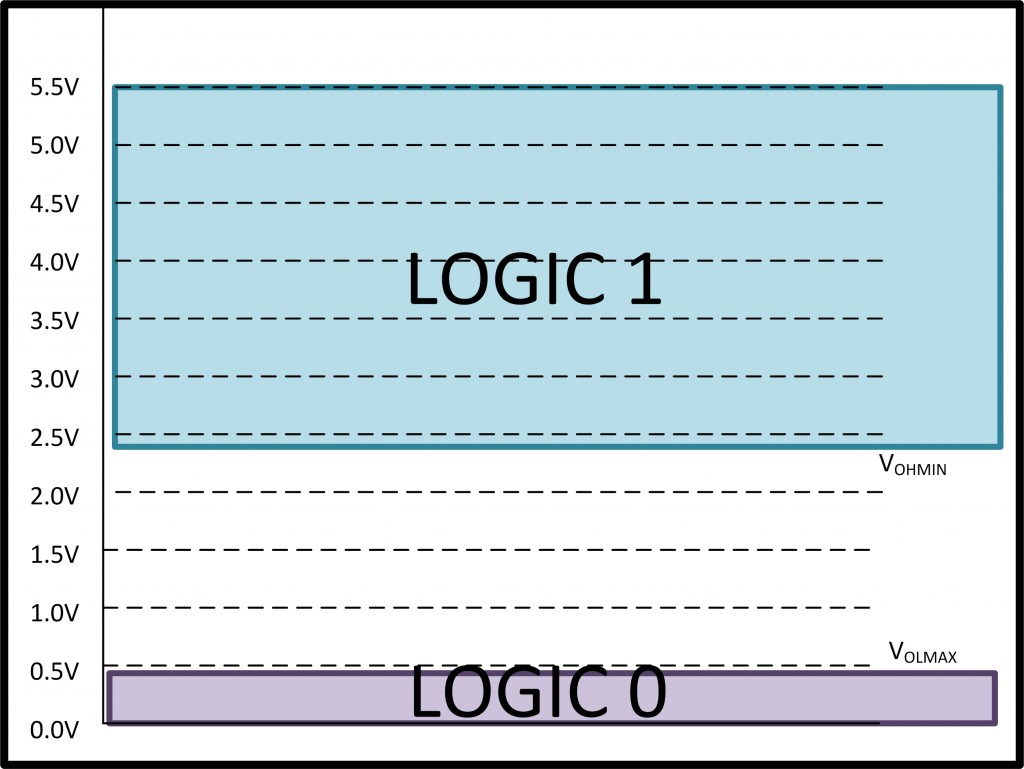### Device Compatibility

When two devices are connected together, we need to determine if the devices are compatible with one another.  Two devices are compatible if both devices can agree upon what minimum voltage level represents logic 1 and what maximum voltage level represents logic 0.

Let’s take an example where two devices are interconnected.  One device will have a IO pin configured as an input and the other will have a IO pin configured as an output.

In order for logic 1 to be interpreted correctly, VOHMIN of the output pin must be greater than the VIHMIN of the input pin.  In order for logic 0 to be interpreted correctly, we need VILMAX to be greater than VOLMAX.  We can view this relationship between the output and input by superimposing the two preceding images.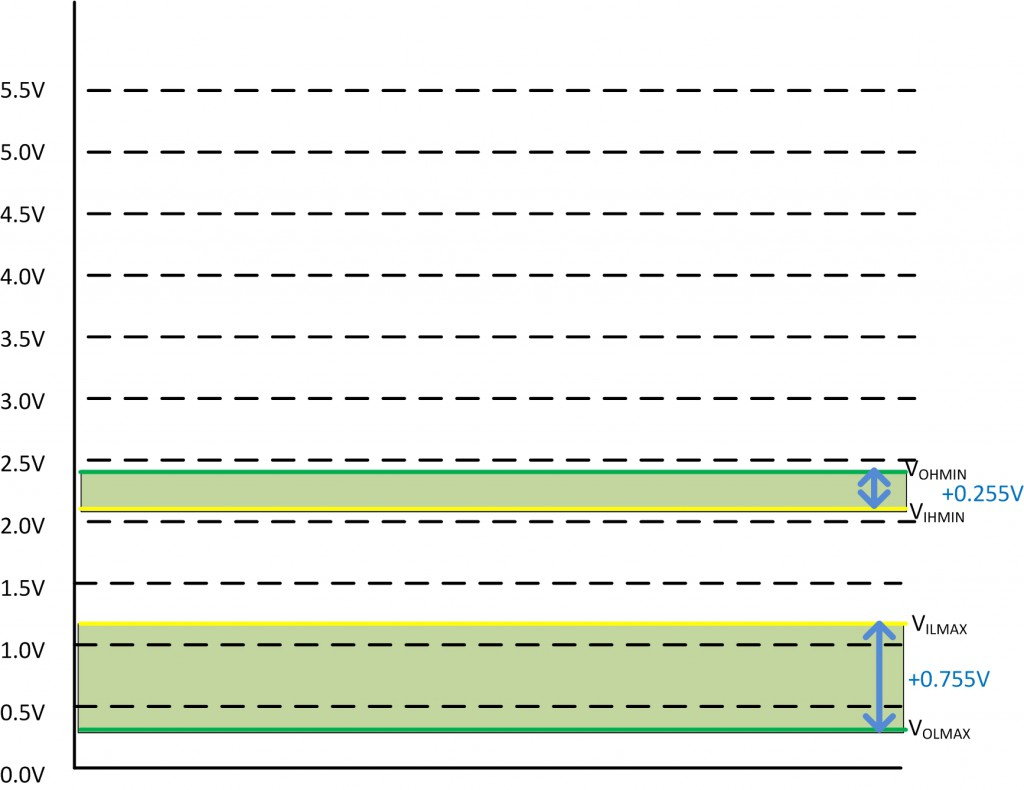When we look at this diagram, we see that there is a gap in between VOHMIN and VIHMIN.  The gap is present because when driving logic 1 as an output, the microcontroller is guaranteed to place a minimum of 2.4V on the output pin.  When configured as an input, a value of 2.145V is required for logic 1.  This gives a gap, or margin, of +0.255V.

The same is true when logic 0 is placed on the output.  The input pin requires that a voltage be less than 1.155V  (VILMAX).  When configured as an output, the microcontroller has a VOLMAX of 0.4V.  This leads to a margin of +0.755V.

When designing an embedded system, the desire is to design a device that will be reliable and operate in a variety of operating conditions.  In certain situations our designs may experience temporary voltage fluctuations that can cause a device to malfunction because the signal violates the maximum or minimum voltage levels for logic 0 or 1.    As a designer, we want to be able to predict how large of a voltage spike our system can tolerate.    We can specify how tolerant our system is by examining the margins between the input and output devices.  These gaps help us to define a term called noise margin.    We take the minimum of the two margins to determine what is called the noise margin for two devices.

Noise Margin = min( VOHMIN – VIHMIN, VILMAX-VOLMAX)

Why do we take the minimum of these two margins?  The answer is that noise may occur when logic 0 or logic 1 is being driven.  The figure below illustrates this by superimposing the same noise profile on both the high and low margins.  If either VIHMIN or VILMAX is violated, our system can malfunction.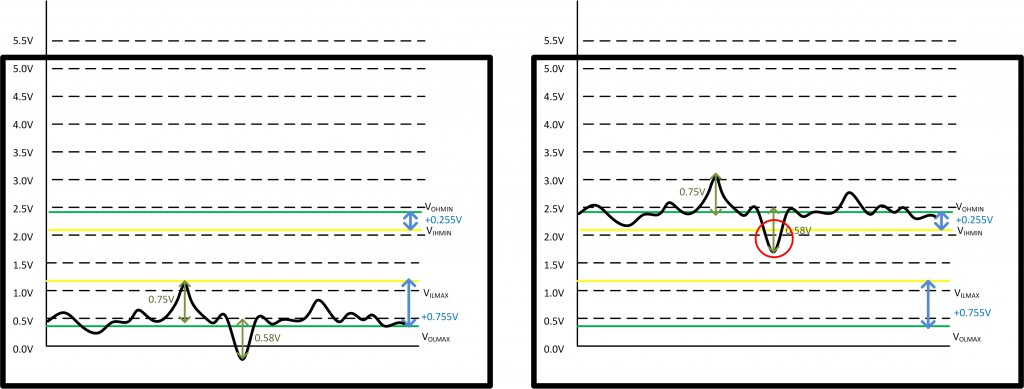Here are a few key points to know

1. Two devices are considered compatible if the both the logic 1 and logic 0 noise margins are positive.
2. For two devices, overall noise margin is the minimum positive noise margin.
3. The system level noise margin is the minimum noise margins between any pair of components.
4. Only calculate the noise margins between devices that are physically connected.

### Example Noise Margin Calculation

In this example, we are going to look at cascaded AND gates and calculate the noise margins.  The gates will be a NC7SZ08P5X.  We will also assume that the supply voltage is 3.3V.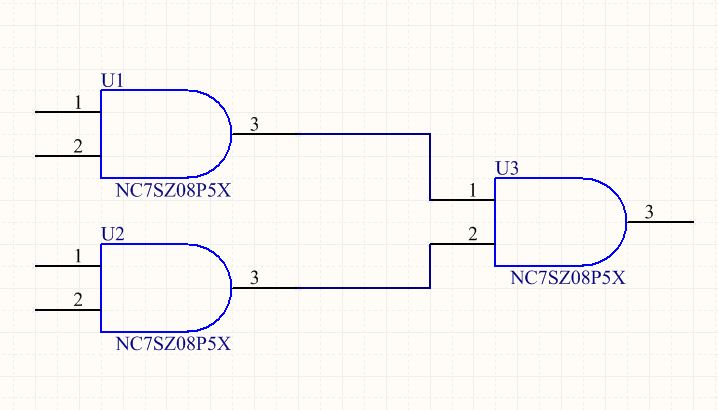The relevant section of the data sheet has been provided below.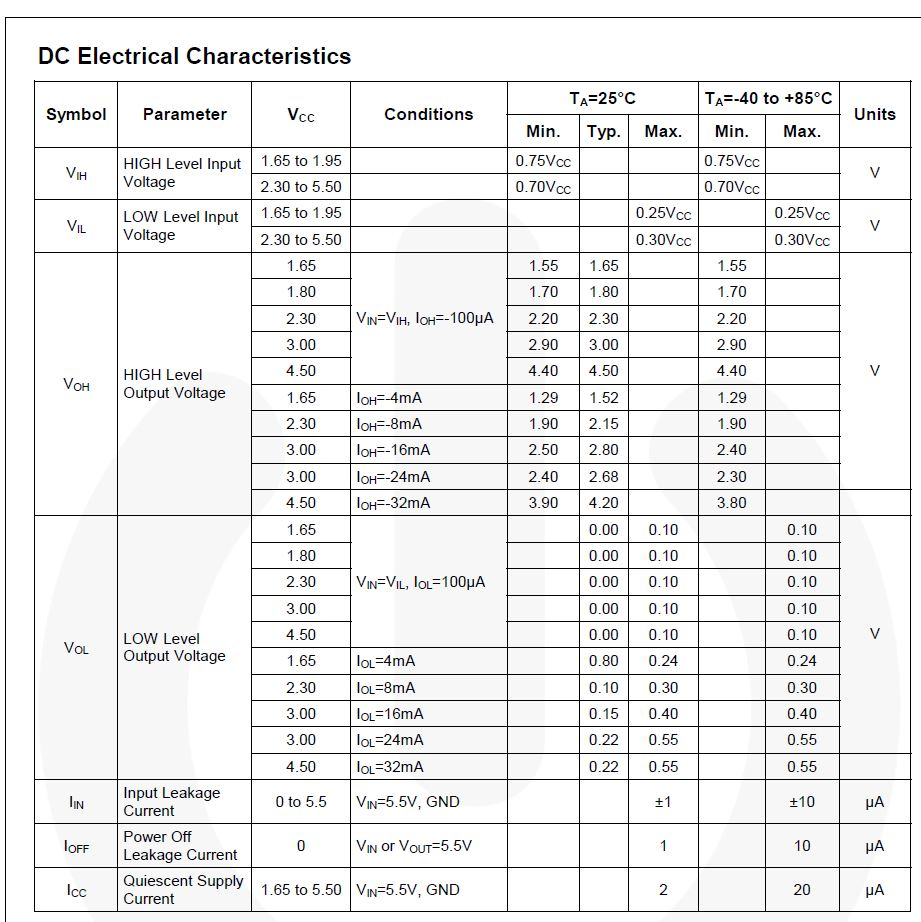U3 has two input pins.  Since both of those pins are being driven by the same part, we can calculate the same noise margin for both pins.  Looking at the input requirements of the NC7SZ08P5X, we can easily calculate VIHMIN and VILMAX

VIHMIN= (3.3V x 0.70) = 2.31V.

VILMAX = (3.3V x 0.30) = 0.99V

The outputs get a little tricky.  There are ten rows to choose from while calculating VOHMIN.  Our supply voltage (3.3V) is not listed, so we will choose to use the 3.0V row since it most closely matches our supply voltage.  But if I look closer, there are still three rows in the VOH row. So which one do we use?

To answer that, we need to understand what the “Conditions” column means.  The conditions column indicates what the value of VOHMIN is when a specified current was being sourced from the pin. We see that for a supply voltage of 3.0V there are entried based on the amount of current being sourced (100µA, 16mA, and 24mA). What we want to do is use the VOHMIN row that most closely matches the current draw for our circuit.

We are driving another NC7SZ08P5X, so we can use the Input Leakage Current (IIN) to determine how much current will be sourced from our output pin when a logic 1 is placed on a pin. In this case, IIN is 10µA, so we will use the entry in the table that most closely matches 10µA.  We have an entry for 100µA that lists 2.9V for VOHMIN. We use the same process for calculating VOLMAX.

VOHMIN= 2.90V

VOLMAX = 0.10V

Using the formula for noise margin, we calculate the noise margin to be
Noise Margin = min( 2.9 – 2.31, 0.99-0.10) = 0.59V

A few observations

1. The lower the supply voltage, the lower the noise margins.
2. A negative current is used to represent current leaving a device.
3. A positive current is used to represent current flowing into a device.
4. An output pin with logic 1 will source current from that pin (negative current).
5. An output pin with logic 0 will sink current from that pin (positive current).
6. As a pin sources more current, the value of VOHMIN starts to decrease.  The result is that noise margins decrease.
7. As a pin sinks more current, the value of VOLMAX increases.  The result is that noise margins decrease.
8. Connecting two devices using TTL level logic results in a current flow in the µAs.  Remember that TTL is transistor to transistor logic.  The leakage current of a transistor is normally in the µA range.
9. Trying to sink/source a current that exceeds the maximum current for an output pin will result in damage to the device.  If you need to drive a high current device, use a FET as a switch.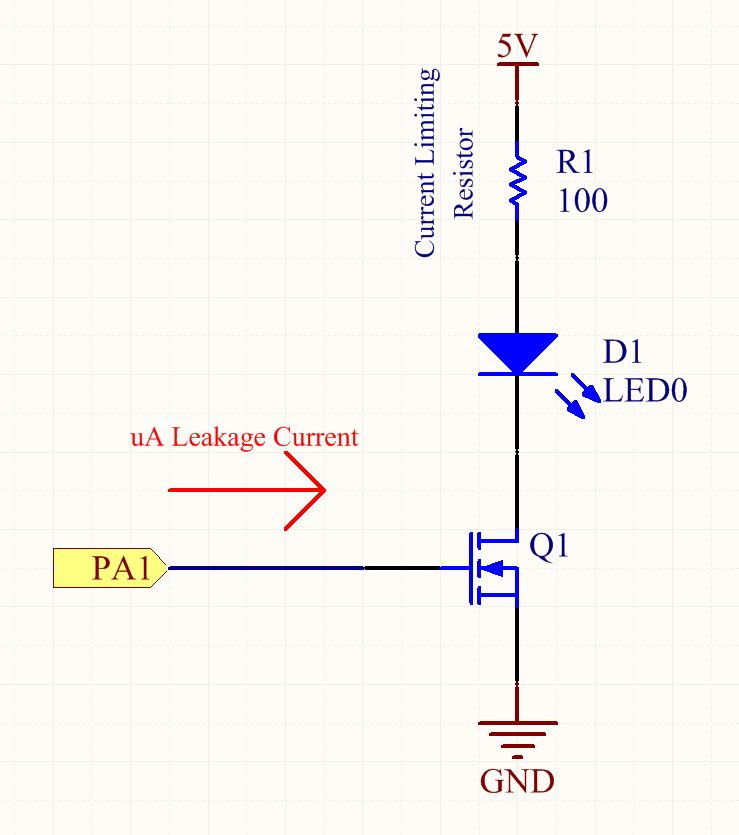The circuit above shows how to drive a LED using a N-Channel MOSFET.  The gate of the FET requires  a few µA or less of current from PA1 (IO pin of microprocessor).  Writing logic 1 to PA1 turns the FET on and allows current to flow through the LED.  The current limiting resistor can then be adjusted to increase/decrease the current flowing through the LED.  The current through the LED can be calculated by subtracting the LED’s forward bias voltage from 5V and dividing by the value of the current limiting resistor.

(5V – VForward Bias)/R1